Simple circuit question

Homework Statement

If a 12 V supply is placed across AD, find effective resistance of whole circuit, current through resistor 3, and voltage reading on resistor 5 with polarity

Homework Equations

Rn= Resistor number n
I= current
V= volts

The Attempt at a Solution

I could not figure if the current would flow from c to b, or b to c, because there is no potential difference across it, and therefore could not solve the problem

Attachments

•simple circuit question.jpg
42.5 KB · Views: 330

help? anyoen?

help? anyoen?

How about drawing it like this...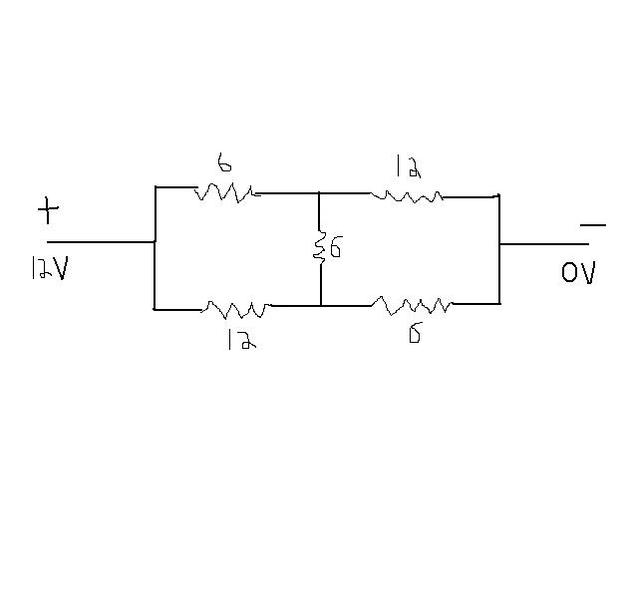How about drawing it like this...

View attachment 25946
yeah, after drawing like that I have no clue how to simplify the diagram....my teacher never even went over it.
I also don't know which direction the current flows through in the middle.
if you could show me how, I would appreciate it so much!

yeah, after drawing like that I have no clue how to simplify the diagram....my teacher never even went over it.
I also don't know which direction the current flows through in the middle.
if you could show me how, I would appreciate it so much!

http://en.wikipedia.org/wiki/Wheatstone_bridge

Now when you look at this, realize that if Vg exists, then you will have current flowing through that middle resistor in your problem. The nice thing about your problem is you know all of the resistors R1-Rx... They are the ones on the "outside".

hey I plugged this equation in: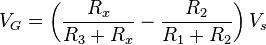but it doesnt work...
you get 4 Volts, when the answer is 6.86 V...

hey I plugged this equation in: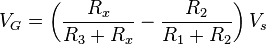but it doesnt work...
you get 4 Volts, when the answer is 6.86 V...

The equation tells you which way the current will flow through the middle resistor. Once you know the direction of the through the various parts of the circuit, you need to use kirchoffs rules. The 5th resistor also comes into play. There is a 5th resistor in the diagram. The way I have it drawn the current should flow down throught the middle resistor.

Last edited:
The equation implies that Vg is always determined by subtracting R2/(R1+R2) from Rx/ (R3+Rx) before multiplying Vs. Why can the equation not be (R2/(R1+R2)- Rx/ (R3+Rx) )Vs instead?
I also dont understand how there is a 5th resister...isn't the 5th resister simply R3??
I'm really confused

And I do get a 6.86V drop across the 12 ohm resistor drawn in your diagram.

This problem requires some time and some calculations. It is difficult for a basic circuit question, but not so if you guys have done Kirchoffs rules. I will put arrows on my diagram that show the flow of current that I figured had to be true due to that link I put up.

ok I think i understand kirschoffs rule better now...just basically plug in all the equations

now how do i determine how much current will enter each branch??Is there a rule for determining this?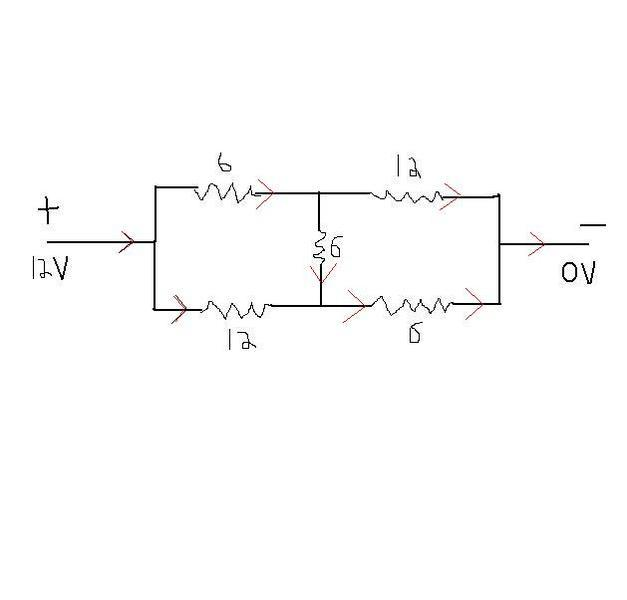ok I think i understand kirschoffs rule better now...just basically plug in all the equations

now how do i determine how much current will enter each branch??Is there a rule for determining this?

Yes. Kirchoffs loop rule helps you determine the voltage drops. But in order to know these you must know the current. This is found using some algebra and variables for the current in various wires. I will do one example of an equation. But I have to label the different parts of the wires. I will do so using the resistor numbers in your problem.

no Im asking about the exact quantity of Amperes that go into each branch...

for example, how do I determine how much amps will go into the initially divided branch that has a 6 ohm resistor on the top, and 12 ohm resistor on the bottom...

no Im asking about the exact quantity of Amperes that go into each branch...

for example, how do I determine how much amps will go into the initially divided branch that has a 6 ohm resistor on the top, and 12 ohm resistor on the bottom...

I understand this.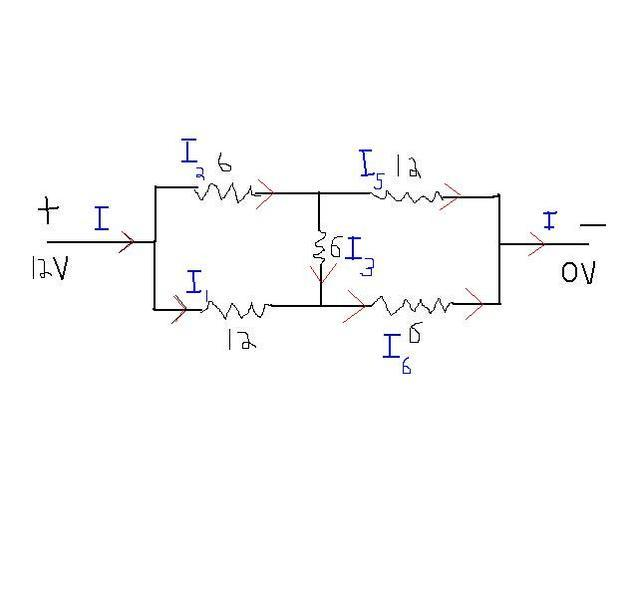So I = I1 + I2
I2 = I3 + I5
I4 = I1 + I3
I = I5 + I4

oops relabel I6 as I4; I will fix in the next picture.

And doing one Kirchoff drop from one end of the battery back to that same point the total voltage drop equals zero: Remember V = R*I

0= 12V - 6 ohms(I2) - 12ohm(I5) this is only one equation. I will now post the path I took in Green.

I get a Voltage drop each time I cross a resistor that has current running through it.

Last edited: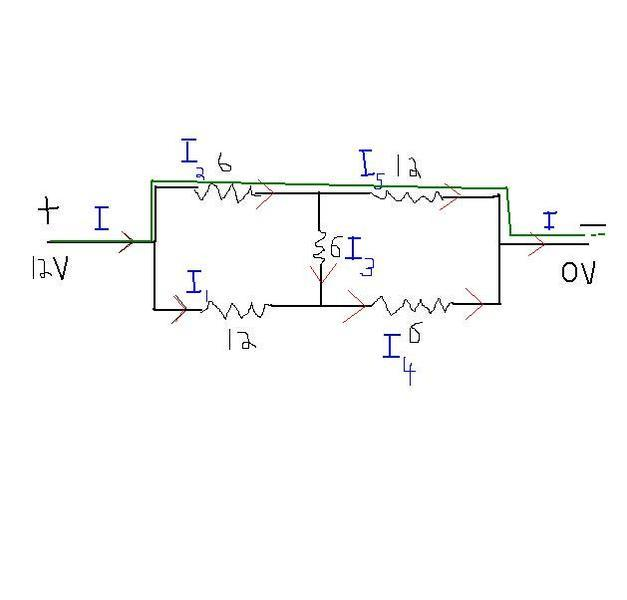From this we can also conclude that I2 = I4 and I1 = I5

Like I said. Its not hard but it takes some algebra work.
Now if you do another path like I did in the post above, you can figure out exact current numbers and thus by I*R get voltage drops.

Last edited:
thx for keeping up with my slow learning

do you get I2 = I4 and I1 = I5, from this system of equations?:
I = I1 + I2
I2 = I3 + I5
I4 = I1 + I3
I = I5 + I4

thx for keeping up with my slow learning

do you get I2 = I4 and I1 = I5, from this system of equations?:
I = I1 + I2
I2 = I3 + I5
I4 = I1 + I3
I = I5 + I4

Yes.

This somewhat of a pain in the rear. When I first looked at it and no one had posted, I thought it might be because its a bit of an algebra/system of equations pain. Some people love these things though. Maybe your teacher does.

!!! I speant my past 30 min trying to solve the system of equations!!!!!!
can u show me how to do this one?
Maybe one example could help me solve all the other questions
]thanks so much

!!! I speant my past 30 min trying to solve the system of equations!!!!!!
can u show me how to do this one?
Maybe one example could help me solve all the other questions
]thanks so much

Start with I1 + I2 = I5 + I4 because both sides equal I...

I tried.. still cant solve it.....

can anoyone else help?

dudes..

Are you sure your teacher did not go over this type of stuff with you?
Some of this physics that involves a number of variables is just a grind it out process. You have to be able to substitute and do some algebra with this stuff. Like I said, I dont find it fun, but ya gotta learn how to do it. Maybe you could go to the math section and they will take you step by step through the algebra. I have read some posts over there and they are very helpful.